• Pytorch实现RNN原理
2021-03-01 21:41:54

# Pytorch实现RNN原理

rnn公式如下。
h t = W h h h t − 1 + W i h X t {{\rm{h}}_t} = {W_{hh}}{{\rm{h}}_{t - 1}} + {W_{ih}}{X_t}
其中 X t {X_t} 表示t时刻的输入序列。Pytorch中RNN的输入 X {X} 大小为[seq, batch_size, embedding]。
所以 X t {X_t} 的大小为[batch_size, embedding]。其中embedding维度是要参与运算的维度，batch_size是要保留的信息。所以一般将 X t {X_t} 的大小写成转置的形式[embedding, batch_size]。

W i h X t {W_{ih}}{X_t} 的结果为[hidden_size, batch_size]。从矩阵的角度来理解就是batchsize个维度为hidden_size的列向量产生都是由原本的序列产生，依赖于自身序列。而与其他的句子无关

上次写的还是有很多小错误，进行了改正

import numpy as np
import torch
from torch import nn
from torch.nn.parameter import Parameter

class Rnn(nn.Module):

def __init__(self, input_size, hidden_size, num_layers, bidirectional=False):
super(Rnn, self).__init__()
self.input_size = input_size
self.hidden_size = hidden_size
self.num_layers = num_layers
self.bidirectional = bidirectional
Wih = [np.random.random((self.hidden_size, self.hidden_size)) for i in range(1, self.num_layers)]
Whh = [np.random.random((self.hidden_size, self.hidden_size)) for i in range(self.num_layers)]
self.Wih, self.Whh = Parameter(torch.tensor(Wih)), Parameter(torch.tensor(Whh))
Wih0 = np.random.random((self.hidden_size, self.hidden_size))
self.Wih0 = Parameter(torch.tensor(Wih0))

def forward(self, x):
'''

:param x: [seq, batch_size, embedding]
:return: out, hidden
'''

# x.shape [sep, batch, feature]
# hidden.shape [hidden_size, batch]
# Whh0.shape [hidden_size, hidden_size]  Wih0.shape [hidden_size, feature]
# Whh1.shape [hidden_size, hidden_size]  Wih1.size  [hidden_size, hidden_size]
if not isinstance(x, torch.Tensor):
raise TypeError('x is not tensor')

out = []
hidden = [np.zeros((self.hidden_size, x.shape)) for i in range(self.num_layers)]

Wih0 = np.random.random((self.hidden_size, x.shape))

# x, hidden, Wih, Whh = torch.from_numpy(x), torch.tensor(hidden), torch.tensor(Wih), torch.tensor(Whh)

hidden = torch.tensor(hidden)
self.Wih0 = Parameter(torch.tensor(Wih0))

time = x.shape
for i in range(time):
hidden = torch.tanh((torch.matmul(self.Wih0, torch.transpose(x[i, ...], 1, 0)) +
torch.matmul(self.Whh, hidden.clone().detach())
))

for i in range(1, self.num_layers):
hidden[i] = torch.tanh((torch.matmul(self.Wih[i-1], hidden[i-1].clone().detach()) +
torch.matmul(self.Whh[i], hidden[i].clone().detach())
))

out.append(hidden[self.num_layers-1])
# 如果list中的元素为tensor，就无法用torch.tensor()转换，会报错

if __name__ == '__main__':
a = torch.tensor([1, 2, 3])
print(torch.cuda.is_available(), type(a))
rnn = Rnn(1, 5, 4)
rnn_office = nn.RNN(1, 5, 4)
# print(list(rnn.parameters()))
input = torch.tensor(np.random.random((6, 3, 1)))
for _ in range(10):
out, h = rnn(input)
# pred = torch.softmax(h.mean(dim=0), dim=1).argmax(dim=1).to(torch.float64)
pred = h.mean(dim=0)
a = rnn.parameters()
# print(rnn)

param = [i for i in a]

label = torch.tensor([1, 2, 3])
criticism = nn.CrossEntropyLoss()
loss = criticism(pred, label)
print(loss.item())
loss.backward()
optimizer.step()

print(rnn_office(input.to(torch.float32)).shape)
print(f'seq is {input.shape}, batch_size is {input.shape} ', 'out.shape ', out.shape, ' h.shape ', h.shape)

# print(sigmoid(np.random.random((2, 3))))
#
# element-wise multiplication
# print(np.array([1, 2])*np.array([2, 1]))



## 分割线

我又将代码稍微调整，使得其可以进行梯度下降计算。

import numpy as np
import torch
from torch import nn

class Rnn(nn.Module):

def __init__(self, input_size, hidden_size, num_layers, bidirectional=False):
super(Rnn, self).__init__()
self.input_size = input_size
self.hidden_size = hidden_size
self.num_layers = num_layers
self.bidirectional = bidirectional

def forward(self, x):
'''

:param x: [seq, batch_size, embedding]
:return: out, hidden
'''

# x.shape [sep, batch, feature]
# hidden.shape [hidden_size, batch]
# Whh0.shape [hidden_size, hidden_size]  Wih0.shape [hidden_size, feature]
# Whh1.shape [hidden_size, hidden_size]  Wih1.size  [hidden_size, hidden_size]

out = []
x, hidden = np.array(x), [np.zeros((self.hidden_size, x.shape)) for i in range(self.num_layers)]
Wih = [np.random.random((self.hidden_size, self.hidden_size)) for i in range(1, self.num_layers)]
Wih0 = np.random.random((self.hidden_size, x.shape))
Whh = [np.random.random((self.hidden_size, self.hidden_size)) for i in range(self.num_layers)]
# x, hidden, Wih, Whh = torch.from_numpy(x), torch.tensor(hidden), torch.tensor(Wih), torch.tensor(Whh)

x = torch.from_numpy(x)
hidden = torch.tensor(hidden)

time = x.shape
for i in range(time):
hidden = torch.tanh((torch.matmul(Wih0, torch.transpose(x[i, ...], 1, 0)) +
torch.matmul(Whh, hidden)
))

for i in range(1, self.num_layers):
hidden[i] = torch.tanh((torch.matmul(Wih[i-1], hidden[i-1]) +
torch.matmul(Whh[i], hidden[i])
))

out.append(hidden[self.num_layers-1])
# 如果list中的元素为tensor，就无法用torch.tensor()转换，会报错

def sigmoid(x):
return 1.0/(1.0 + 1.0/np.exp(x))

if __name__ == '__main__':
a = torch.tensor([1, 2, 3])
print(torch.cuda.is_available(), type(a))
rnn = Rnn(1, 5, 4)
input = np.random.random((6, 2, 1))
out, h = rnn(input)
print(f'seq is {input.shape}, batch_size is {input.shape} ', 'out.shape ', out.shape, ' h.shape ', h.shape)
# print(sigmoid(np.random.random((2, 3))))
#
# element-wise multiplication
# print(np.array([1, 2])*np.array([2, 1]))


## 分割线

首先说明代码只是帮助理解，并未写出梯度下降部分，默认参数已经被固定，不影响理解。代码主要实现RNN原理，只使用numpy库，不可用于GPU加速。

import numpy as np

class Rnn():

def __init__(self, input_size, hidden_size, num_layers, bidirectional=False):
self.input_size = input_size
self.hidden_size = hidden_size
self.num_layers = num_layers
self.bidirectional = bidirectional

def feed(self, x):
'''

:param x: [seq, batch_size, embedding]
:return: out, hidden
'''

# x.shape [sep, batch, feature]
# hidden.shape [hidden_size, batch]
# Whh0.shape [hidden_size, hidden_size]  Wih0.shape [hidden_size, feature]
# Whh1.shape [hidden_size, hidden_size]  Wih1.size  [hidden_size, hidden_size]

out = []
x, hidden = np.array(x), [np.zeros((self.hidden_size, x.shape)) for i in range(self.num_layers)]
Wih = [np.random.random((self.hidden_size, self.hidden_size)) for i in range(1, self.num_layers)]
Wih.insert(0, np.random.random((self.hidden_size, x.shape)))
Whh = [np.random.random((self.hidden_size, self.hidden_size)) for i in range(self.num_layers)]

time = x.shape
for i in range(time):
hidden = np.tanh((np.dot(Wih, np.transpose(x[i, ...], (1, 0))) +
np.dot(Whh, hidden)
))

for i in range(1, self.num_layers):
hidden[i] = np.tanh((np.dot(Wih[i], hidden[i-1]) +
np.dot(Whh[i], hidden[i])
))

out.append(hidden[self.num_layers-1])

return np.array(out), np.array(hidden)

def sigmoid(x):
return 1.0/(1.0 + 1.0/np.exp(x))

if __name__ == '__main__':
rnn = Rnn(1, 5, 4)
input = np.random.random((6, 2, 1))
out, h = rnn.feed(input)
print(f'seq is {input.shape}, batch_size is {input.shape} ', 'out.shape ', out.shape, ' h.shape ', h.shape)
# print(sigmoid(np.random.random((2, 3))))
#
# element-wise multiplication
# print(np.array([1, 2])*np.array([2, 1]))

更多相关内容
• 1.什么是RNN RNN（Recurrent Neural Network）循环神经网络，是用来专门处理序列数据的神经网络。百度百科关于时间序列数据的定义是这样的：时间序列数据是指在不同时间点上收集到的数据，这类数据反映了某一事物、...

1.什么是RNN

RNN（Recurrent Neural Network）循环神经网络，是用来专门处理序列数据的神经网络。百度百科关于时间序列数据的定义是这样的：时间序列数据是指在不同时间点上收集到的数据，这类数据反映了某一事物、现象等随时间的变化状态或程度。这是时间序列数据的定义，当然这里也可以不是时间，比如文字序列，但总归序列数据有一个特点——后面的数据跟前面的数据有关系。

RNN的出现是为了解决全连接神经网络不能联系上下文去训练模型的缺点。有一个NLP很常见的问题命名实体识别，举个例子，有下面两句话：

第一句：I like eating apple!

第二句：The Apple is a great company!

现在我们要给apple打标签，假设现在有很多已经标记好的数据供我们训练模型。假如我们使用全连接神经网络，把apple对应的特征向量输入到网络中，那么输出结果中我们想让正确的标签概率值最大，来训练模型，我们的训练集中有的apple的标签是苹果有的是公司，这将导致训练的准确度取决于训练集中哪个标签的个数比较多，这显然是我们不愿看到的。

但如果让我们去判断，那么一定是不能只看apple这个词的，是要联系上下文的。但全连接神经网络是不能做到这一点的，于是就有了循环神经网络。

2.RNN为什么能处理序列数据

这是RNN的结构决定的。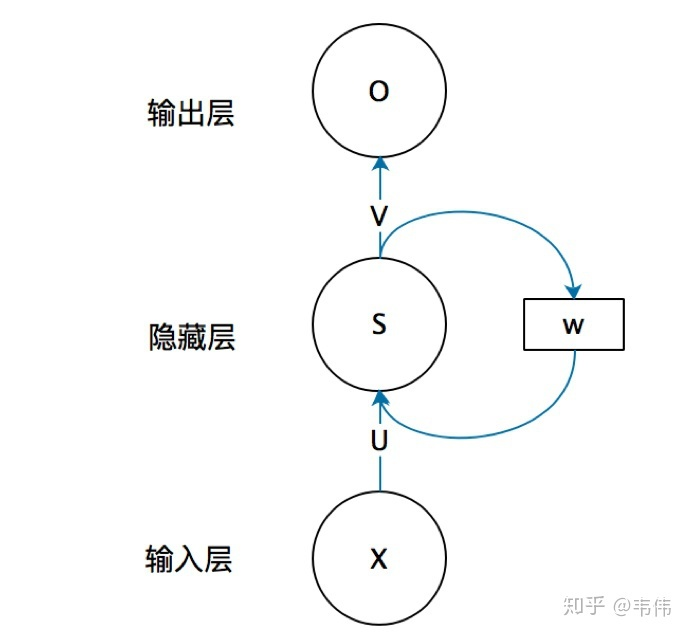这是网上很经典的一张RNN的结构图，他每层不只有一个神经元，不看矩阵w,把他展开可以是这样的：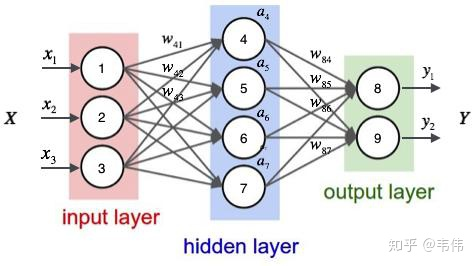这就是一个全连接神经网络。u就是一个3×4的矩阵，s的维度是4，v是4×3的矩阵。

如果按照第一张图的w展开他也可以变成这样：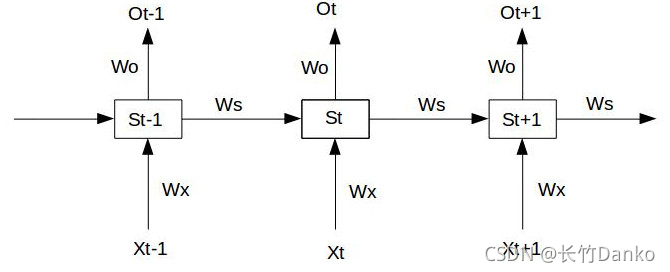这是不同时刻输入x对应输出的图，这体现了RNN的很重要的一个思想：权重共享。即不同时刻输入的x每次使用的权重矩阵是一样的，每一部分都是如此。

有了这个图我们就可以解释RNN是如何处理序列数据的了，其关键之处在于循环核，也就是图中的Ws矩阵，他能记住上次隐藏层输出的特征，并将他传递给下一次输入。

我们来看RNN的前向传播的公式：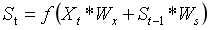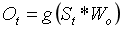隐藏层的输出不仅与本次输入有关还和上一时刻隐藏层的输出有关，这样网络便能记住不同时刻的特征，于是他便能处理序列数据。

3.RNN的反向传播

RNN对于每个时刻输入的x都会产生一个损失函数值，总的损失函数是每个时刻的叠加，我们只以某个时刻为例来推导他的反向传播公式，实际是用各个时刻的损失函数的和来求梯度的。

以第三幅图为例，在t时刻（使用平方损失函数），假设t=3。

损失函数为：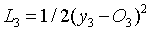y为真实的输出，各个参数的梯度为：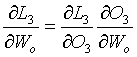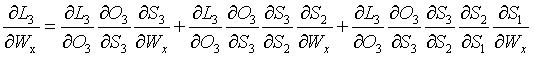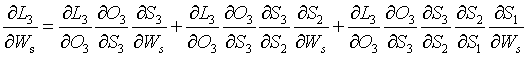以Wx的梯度为例，因为S3不仅和本层输入有关，还和S2有关而S2又和S1有关，所以Wx的梯度会有三部分，Ws也是一样。

于是我们便可以推出任意时刻Wx的梯度为：Ws与之类似，只不过把Ws替换一下，这样便可以用梯度下降法进行更新参数了。

4.RNN存在的问题

梯度消失和梯度爆炸是RNN存在的两个问题，他们是如何产生的哪？

我们看上面推出的任意时刻Wx的梯度就会发现中间的连乘的部分每次Sj对Sj-1求导都会产生一个Ws和一项损失函数的导数(因为先要对损失函数整体求导)，如果连乘的项过多那么就会有Ws的n次方的项产生如果Ws大于或小于1就会让这个梯度本身很大或很小，也就是产生梯度爆炸或梯度消失。

当然这里说的消失并不是整个梯度都没有了，而是当t较大时(就是后面输入的x)的梯度没有了，这也限制了RNN的学习能力，就是他不能学习远距离的依赖关系。

我们也不能忽略了求导过程中损失函数的导数这一项，他的大小同样会加重梯度消失或爆炸。

如何解决梯度消失或梯度爆炸？

梯度消失：可以使用不同的损失函数比如ReLU,他求导后的值总为1(对于大于0的值)，还有leakrelu、elu函数等，或者改变RNN本身的结构，这就是接下来我们要学习的LSTM(Long Short Term Memory, 长短期记忆网络 )和GRU(Gated Recurrent Unit networks,门控循环单元网络）

梯度爆炸：梯度裁剪，权值正则化。

参考文章：

史上最详细循环神经网络讲解（RNN/LSTM/GRU） - 知乎马上就要入职了，担心自己啥都忘了被领导爆锤，在此复习一下之前学过的知识。我相信每次的学习整理都会对自己更加深刻理解这些知识有很大的帮助，同时也希望更多的人看了我的文章有所收获。今天先来复习一下，循环…https://zhuanlan.zhihu.com/p/123211148

通俗易懂的RNN_长竹Danko的博客-CSDN博客_rnnRNN，LSTM，GRURNN循环核介绍：参数时间共享，循环层提取时间信息。功能快捷键合理的创建标题，有助于目录的生成如何改变文本的样式插入链接与图片如何插入一段漂亮的代码片生成一个适合你的列表创建一个表格设定内容居中、居左、居右SmartyPants创建一个自定义列表如何创建一个注脚注释也是必不可少的KaTeX数学公式新的甘特图功能，丰富你的文章UML 图表FLowchart流程图导出与导入导出导入RNNRNN（Recurrent Neural Network）是一类用于处理序列数据的神经网络。首先我
https://blog.csdn.net/qq_39439006/article/details/121554808

展开全文机器学习
• RNN介绍： RNN与HMM的区别： 主要是隐状态，HMM相当于是onehot编码，每个观测状态是由某一个隐状态决定的，其他都为0.属于局部最优解，稀疏。 而RNN的隐状态相当于词向量表示（分布式表示），每个观测状态是由...

# RNN介绍：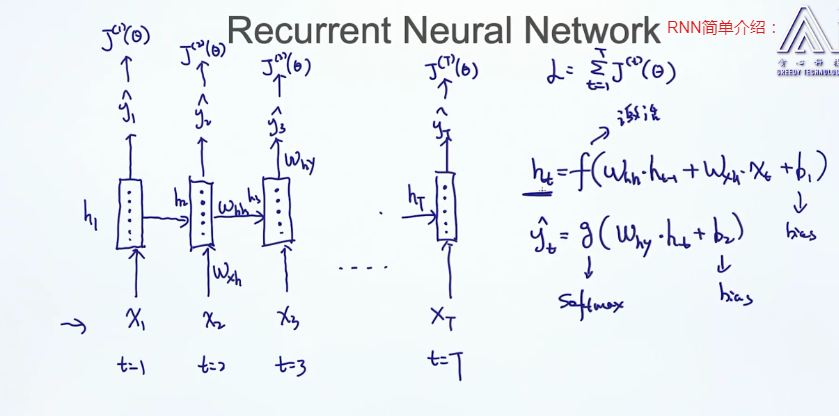# RNN与HMM的区别：

主要是隐状态，HMM相当于是onehot编码，每个观测状态是由某一个隐状态决定的，其他都为0.属于局部最优解，稀疏。

而RNN的隐状态相当于词向量表示（分布式表示），每个观测状态是由好多隐状态作用生成的，密集。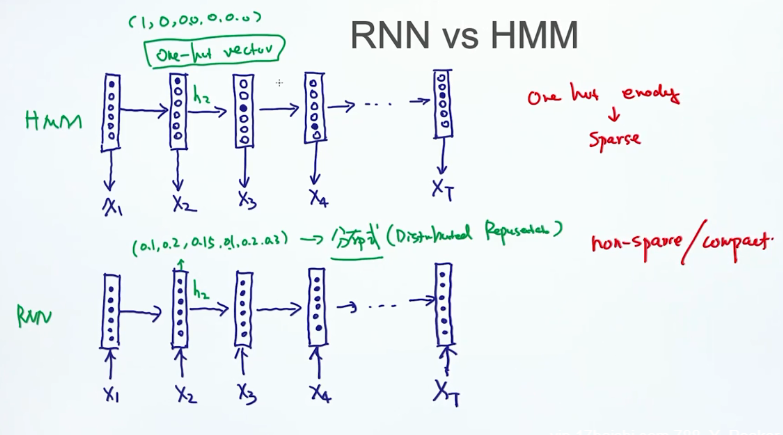递归神经网络适合语言模型，因为后边的输出会受前边输入的影响，所以一个句子后边的词的生成是联合概率。但存在的问题是

梯度消失，随着句子加长，生成后边的词时，越远的词的作用会越来越小，所以有了LSTM。

每一个时间点的输出都是一个长度为V（词典里词个数）的向量，通过交叉熵损失函数计算出每个输出词的损失，然后再累加作为损失函数，优化这个损失函数得到训练参数。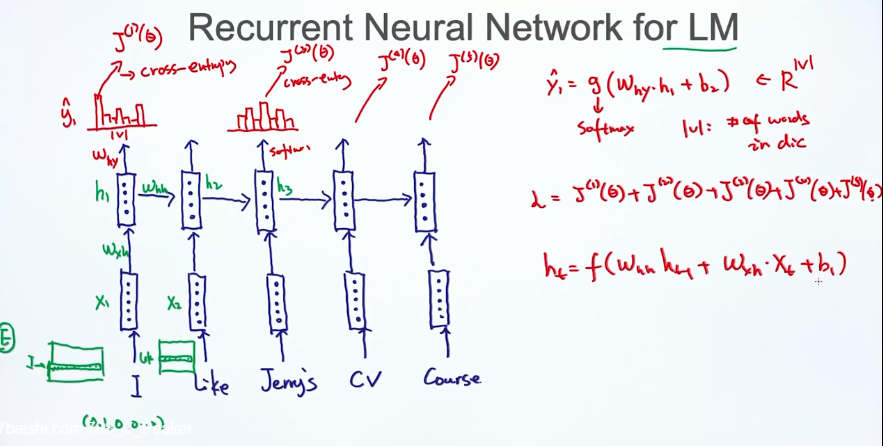RNN的问题是梯度消失与爆炸。

梯度爆炸容易解决：设置一个阈值，当结果大于这个值时，将其减小。

梯度消失不好解决：可以用LSTM，激活函数换成RELU。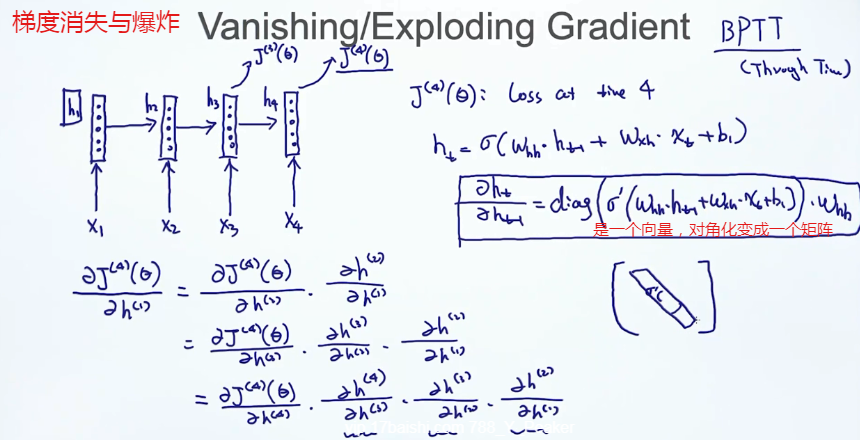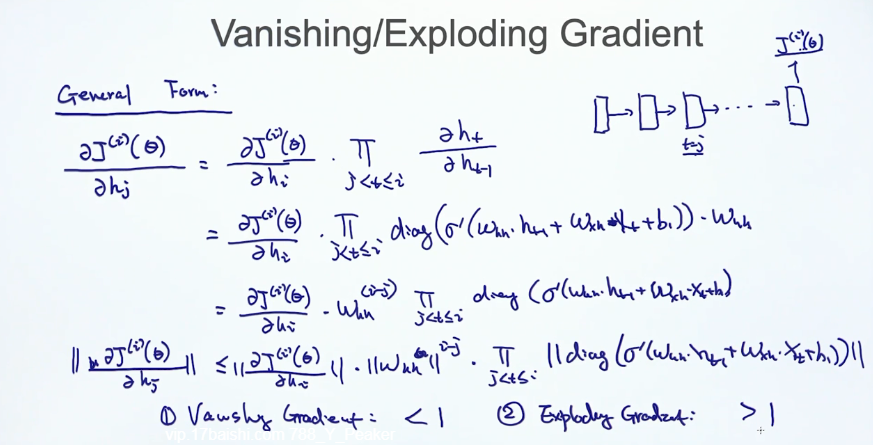# LSTM的提出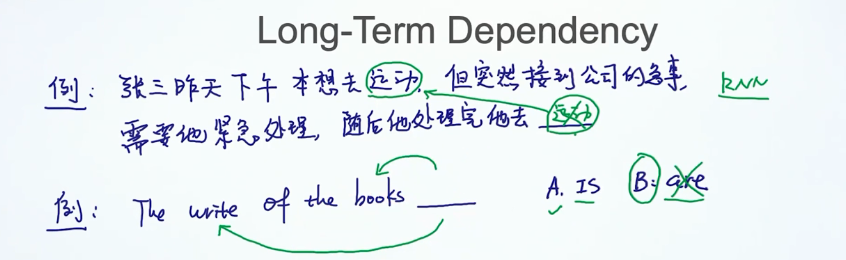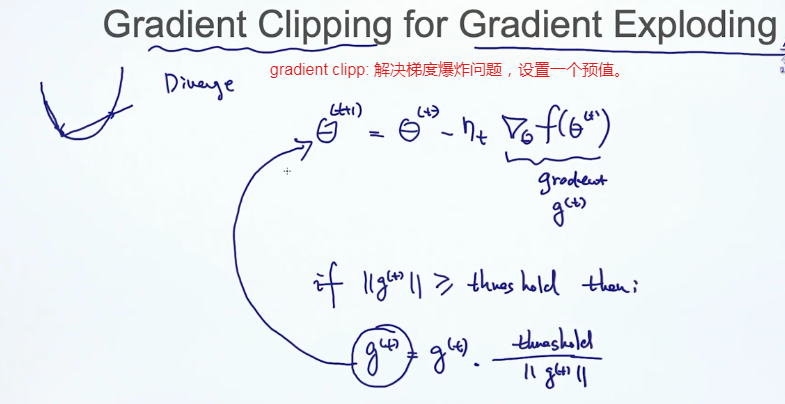LSTM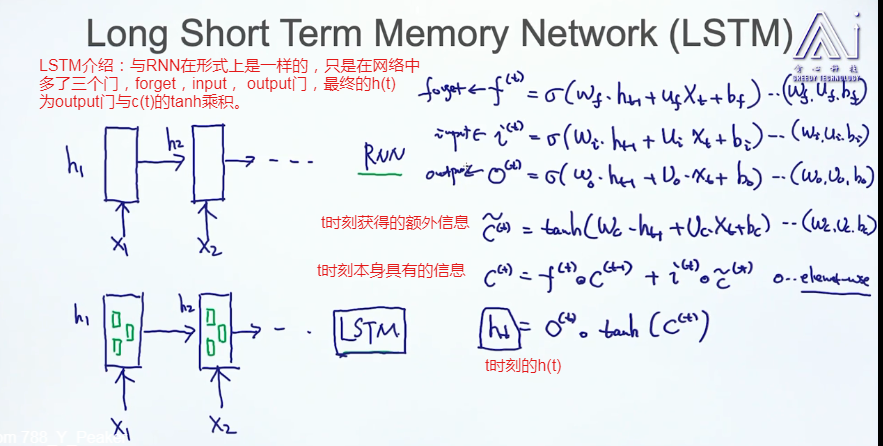sigmoid函数选择更新内容，tanh函数创建更新候选。

这篇博客对RNN有了很详细的介绍，在此引用作为参考。

https://blog.csdn.net/zhaojc1995/article/details/80572098

RNN的一些变种： 看图说话，文本分类，机器翻译，命名实体标签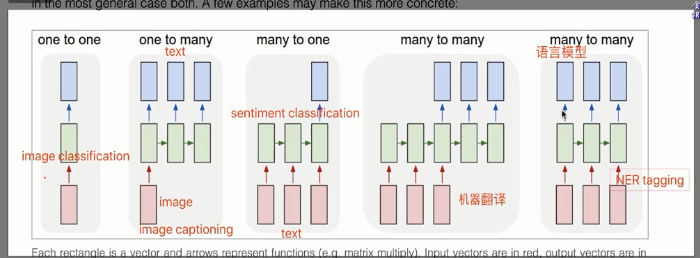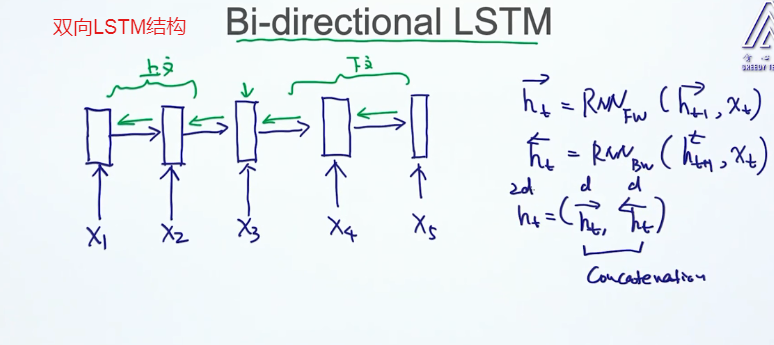# GRU：结构、参数比RNN简单，效果有时并不差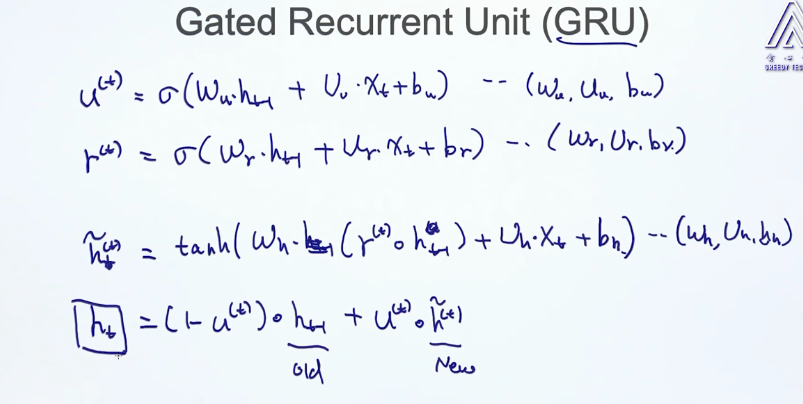展开全文自然语言处理
• 传统的神经网络长度方面不适用（传统的都是等长的），传统的神经网络不能联系上下文。所以处理sequence data 需要新的模型 RNN

传统的神经网络长度方面不适用（传统的都是等长的），传统的神经网络不能联系上下文。所以处理sequence data 需要新的模型 RNN。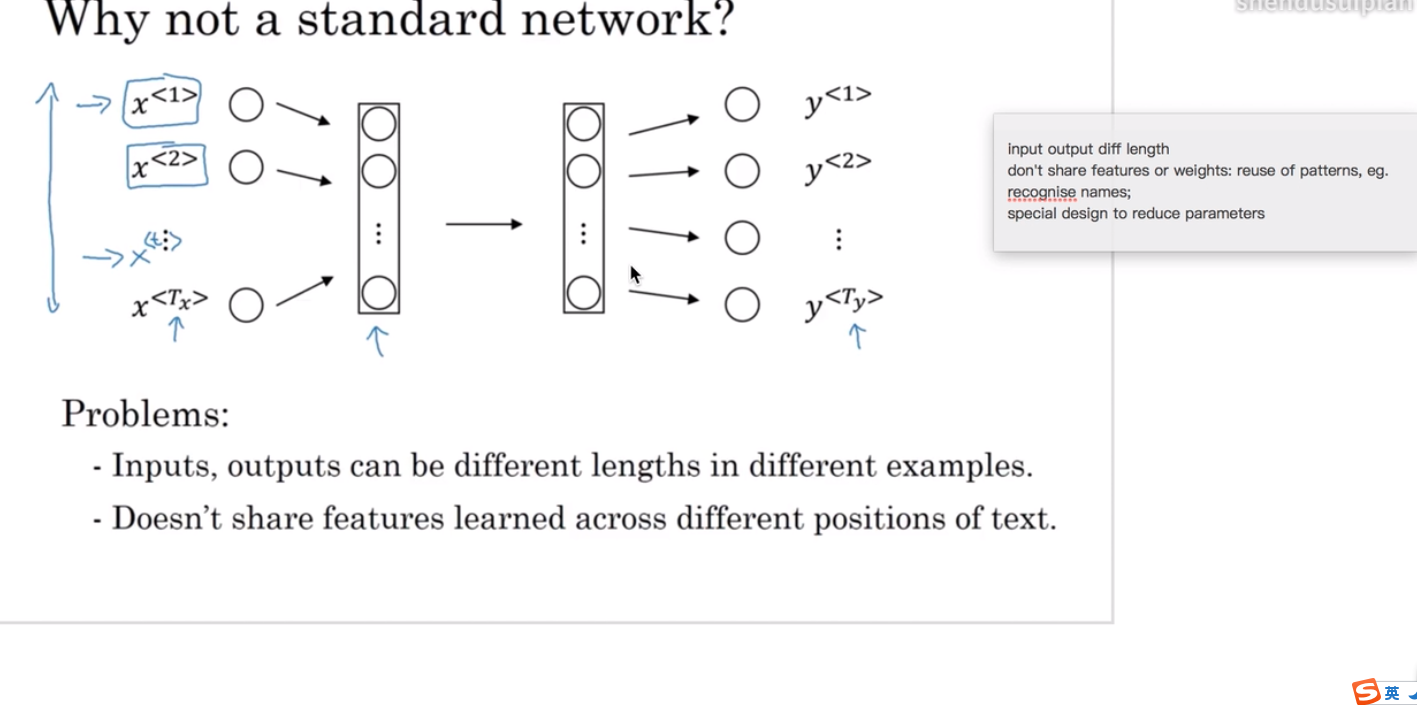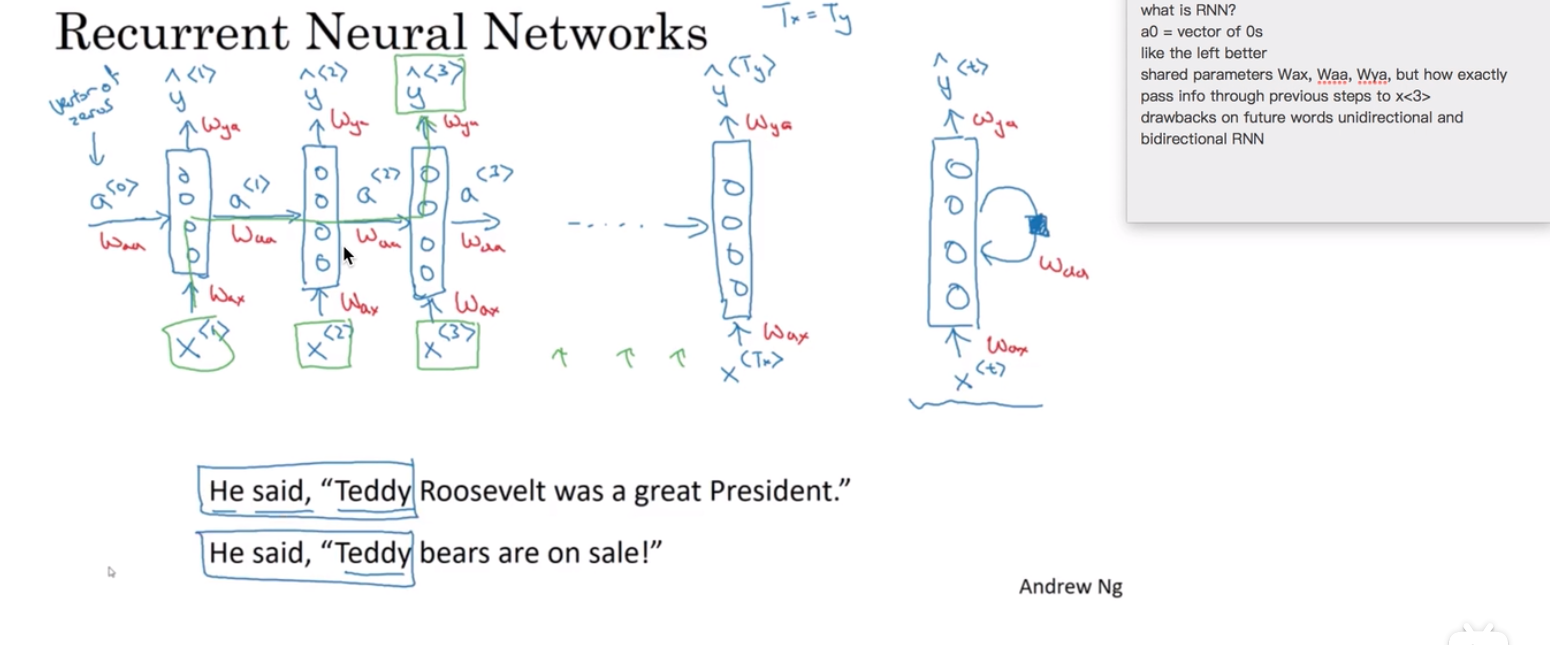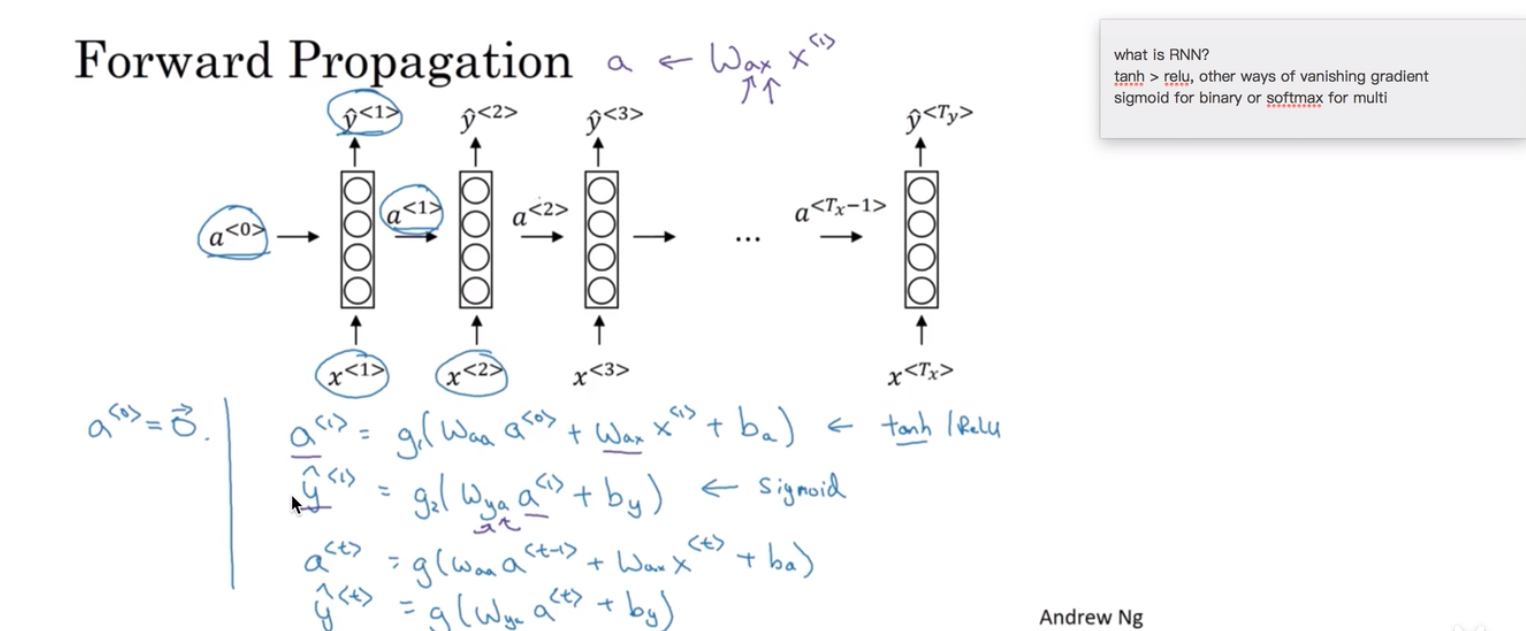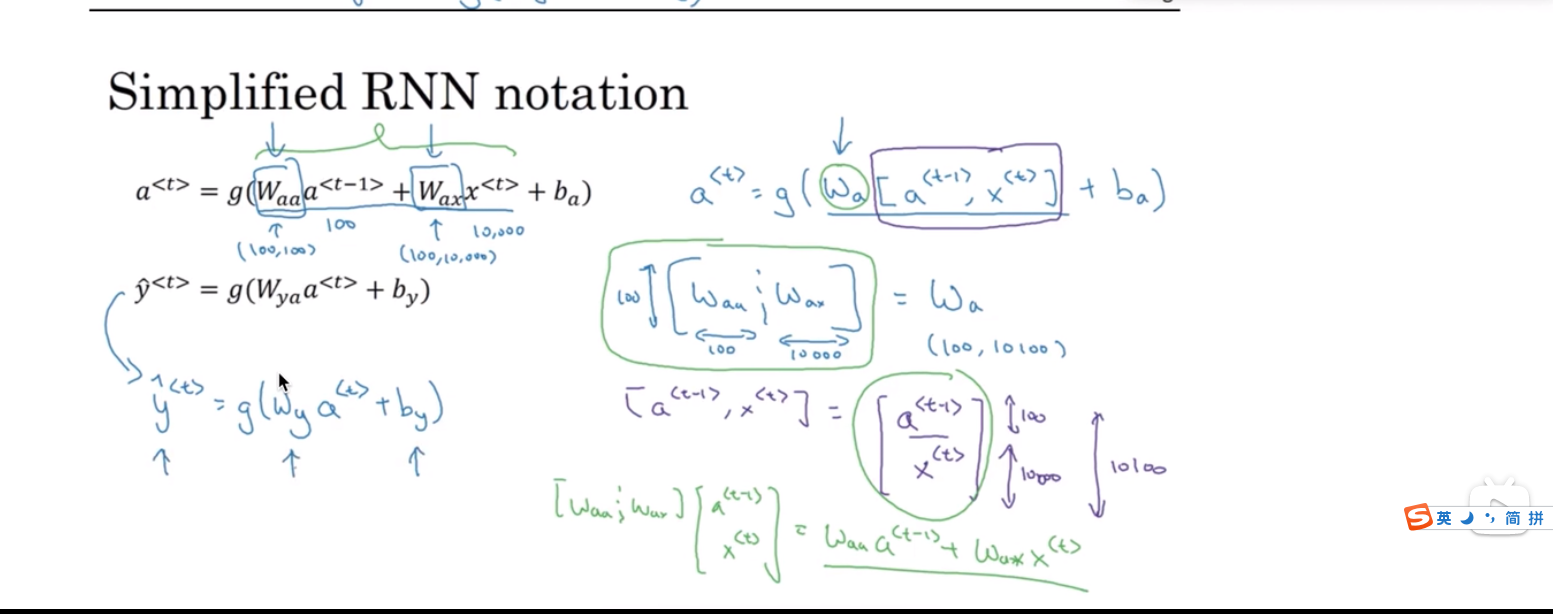展开全文• RNN原理的结构图如下面所示： 由上图可知以下公式，其中 V 是输出层权重矩阵，g 是激活函数；U 是输入x的权重矩阵，W 是上一次的值 st-1 作为这一次的输入的权重矩阵，f 是激活函数。 2 双向rnn循环神经网络 其...自然语言处理 深度学习 神经网络
• ## RNN原理小结

万次阅读 2018-05-12 19:24:35
循环神经网络(RNN, Recurrent Neural Networks)介绍   这篇文章很多内容是参考：http://www.wildml.com/2015/09/recurrent-neural-networks-tutorial-part-1-introduction-to-rnns/，在这篇文章中，加入了一些新的...
• ## 双向RNN原理

千次阅读 2020-05-05 22:44:01
我们之前已经了解了RNN中的GRU和LSTM。 怎么样才能进一步优化RNN这样的模型呢？就是使用双向RNN，它能使得我们在序列的某点处，不仅获取之前的信息，还能获取将来的信息。 将来的信息是什么意思呢？为什么根据...算法 人工智能 机器学习
• 这是我看过的最好的RNN原理图，理解这个就可以了。
• 为什么要使用RNN前馈神经网络前馈神经网络是一种最简单的神经网络，是目前应用最广泛、发展最迅速的人工神经网络之一。各神经元分层排列，第一层是输入层；然后是隐藏层，其中隐藏层可能会有多层；最后一层为输出层...
• 一、循环神经网络（RNN原理通俗解释 在图像处理中，目前做的最好的是CNN 自然语言处理中，表现比较好的是RNN 既然我们已经有了人工神经网络和卷积神经网络，为什么还要循环神经网络？ 原因很简单，无论是卷积...人工智能 深度学习
• 1.首先参考CNN和RNN文本分类对比：http://blog.csdn.net/baoyan2015/article/details/64438530http://blog.csdn.net/u010223750/article/details/51437854​2.RNN做文本分类，相当于把每个词作为一个时间节点，把词...
• 目录引言一、原理《深度学习》中关于循环和递归网络笔记1、简介2、展开计算图3、循环神经网络4、莫烦python1、简介二、tensorflow实现三、总结 引言 因为前段时间在跟B站学习强化学习，其中提到过循环神经网络，于是...tensorflow lstm 人工智能 机器学习
• 这篇文章主要介绍了numpy实现RNN原理实现，文中通过示例代码介绍的非常详细，对大家的学习或者工作具有一定的参考学习价值，需要的朋友们下面随着小编来一起学习学习吧 首先说明代码只是帮助理解，并未写出梯度...numpy
• (手误写错了) f,g 为激活函数 （1）无RNN的处理模式，从固定大小的输入到固定大小的输出（例如图像分类）。 （2）序列输出（例如，图像字幕拍摄图像并输出单词句子）。 （3）序列输入（例如，情感分析，其中给定句子...
•人工智能 深度学习 NLP
• 最近正在看递归神经网络，看了网上很多博文，算是鱼龙混杂，并且基本都是使用Python实现，要不就是使用Matlab中的函数库newelm()等。对于使用Matlab的同学，甚为不方便。所以我将结合实例，使用matlab语言，完成...Elman 递归神经网络 MATLAB BPTT
• 曝光一个垃圾博客纯粹是胡说八道赚取流量的：https://www.jianshu.com/p/2a688b1eaeb3 错误1： rnn原理：https://blog.csdn.net/qq_39422642/article/details/78676567python pytorch 深度学习
• 正文共1523张图，3张图，预计阅读时间8分钟。1、简介Char-RNN，字符级循环神经网络，出自于Andrej Karpathy写的The Unreasonable E...
• 为了解决RNN存在的梯度消失问题，科学家们花费7年时间，分析出了LSTM（长短时记忆网络），解决了RNN的问题，此前，说人们通过RNN取得了显著的成果，这些成果基本上都是使用LSTM实现的。这足以表明LSTM的强大。 ...
• RNN Xt表示t时刻的输入;ot表示t时刻的输出,St表示t时刻的记忆X_t 表示t时刻的输入;o_t表示t时刻的输出,S_t表示t时刻的记忆Xt​表示t时刻的输入;ot​表示t时刻的输出,St​表示t时刻的记忆 St=f(U∗Xt+W∗St−1)S_...深度学习 机器学习
• RNN 是一种时序链特征的循环神经网络。 主要应用于： ① 自然语言处理(NLP): 主要有视频处理, 文本生成, 语言模型, 图像处理 ② 机器翻译, 机器写小说 ③ 语音识别 ④ 图像描述生成 对于时序链的特征预测,最...算法
• 从本专栏开始，作者正式研究Python深度学习、神经网络及人工智能相关...这篇文章将详细讲解循环神经网络RNN原理知识，并采用TensorFlow实现手写数字识别的RNN分类案例及可视化呈现。基础性文章，希望对您有所帮助！人工智能 Python Keras 循环神经网络
• 对于具有时间维度的数据，比如阅读的文本、说话时发出的语音信号、随着时间变化的...下面就来详细说明其原理RNN的结构 基础的神经网络只在层与层之间建立了权连接，而 RNN 则在此基础上在层之间的神经元之间也建机器学习
• ## 循环神经网络（RNN）原理通俗解释

万次阅读 多人点赞 2017-11-30 15:15:37
2.RNN的网络结构及原理 3.RNN的改进1：双向RNN 4.RNN的改进2：深层双向RNN 4.1 Pyramidal RNN 5.RNN的训练-BPTT 6.RNN与CNN的结合应用：看图说话 7.RNN项目练手 1.RNN怎么来的？ 循环神经网络的应用场景...神经网络
• Keras 的RNN实现有三种，分别为： SimpleRNN GRU LSTM 一个最简单的RNN数学公式表达就是： Yn+1=W.∗X+U.∗Yn+b Y_{n+1} = W.*X + U.*Y_{n}+b Yn+1​=W.∗X+U.∗Yn​+b 我们就是要训练Trainning data的......